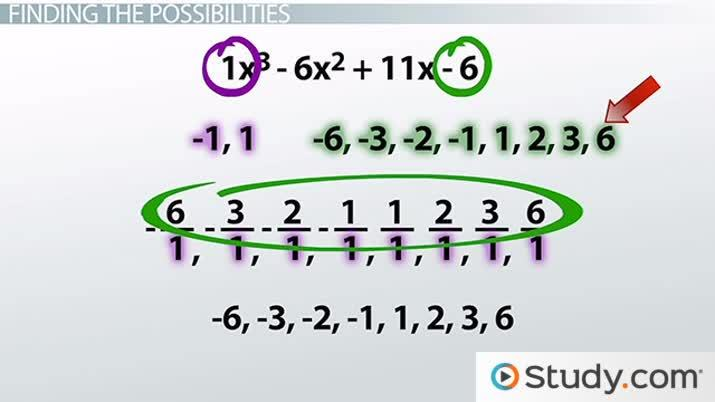# Rational Root Theorem Overview & Examples

Courtney Hitchings, Yuanxin (Amy) Yang Alcocer
• Author
Courtney Hitchings

Courtney has 6+ years of high school math education experience. She received her BA in Math Secondary Education at the University of Delaware and her M.Ed in Curriculum and Instruction from Concorida University.

• Instructor
Yuanxin (Amy) Yang Alcocer

Amy has a master's degree in secondary education and has been teaching math for over 9 years. Amy has worked with students at all levels from those with special needs to those that are gifted.

Discover what the rational root theorem is and what rational roots are. Using examples, understand how the rational root theorem is helpful in finding possible and exact roots of a polynomial. Updated: 01/31/2022

Show

## What is the Rational Root Theorem?

The Rational Root Theorem is used in math to find the possible rational roots of a polynomial function, most specifically when the function is not factorable. These rational roots can also be called: x-intercepts, zeros or solutions. To solve for them set the polynomial equal to 0, as y=0 on our x-axis. In order to use the Rational Root Theorem, it is important to understand the basics of a polynomial function. A polynomial function is defined as a function involving only positive integer powers of x with terms combined with addition and subtraction. A polynomial is in standard form if the terms are in order from highest exponent to lowest. For example, {eq}3x^3 + 2 - 4x^5 {/eq} in standard form is {eq}-4x^5 + 3x^3 +2 {/eq}. The leading coefficient in a polynomial is the first term only if the polynomial is in standard form, if not, then look to the term with the highest exponent. The constant term of a polynomial is the only term that does not have a variable. In the example before, -4 is the leading coefficient and 2 is the constant. Lastly, a rational number is a number that can be expressed as the quotient or fraction of two integers. This means that numbers such as {eq}\pi {/eq} are not rational because they are repeating decimals.

The Rational Root Theorem states that for a polynomial equation with integer coefficients to have a rational solution, that solution must be represented by the a factor of the constant (denoted by the variable p) divided by a factor of the leading coefficient (denoted by the variable q). This means, the rational root theorem results in finding all possible solutions from a polynomial by listing all of the factors of both the leading coefficient and constant and representing them as a fraction. This is represented by listing the possible roots as {eq}\frac{+}{-} \frac{p}{q} {/eq}

### Understanding the Rational Root Theorem

The Rational Root Theorem (RRT) is used to help in a few different situations

• Solving a polynomial with more than 4 terms
• Solving a polynomial with a degree 3 or higher that is not factorable
• Finding out where to start on a problem

Note that the RRT only provides the possible solutions to a polynomial. There are still more steps needed to be able to confirm a root.An error occurred trying to load this video.

Try refreshing the page, or contact customer support.

Coming up next: How to Evaluate a Polynomial in Function Notation

### You're on a roll. Keep up the good work!

Replay
Your next lesson will play in 10 seconds
• 0:03 Rational Roots Theorem
• 2:09 Possible Solutions
• 2:40 Finding the Possibilities
• 4:15 Finding a Solution
• 5:45 Lesson Summary
Save Save

Want to watch this again later?

Timeline
Autoplay
Autoplay
Speed Speed

## Rational Root Theorem Examples

Here are a few examples to show how the Rational Root Theorem is used.

### Example 1: Finding Rational Roots

Using the polynomial {eq}f(x) = x^3 + x^2 + x - 3 {/eq} answer the following questions.

1) Find the possible rational roots of the polynomial.

First identify that our constant (p) is -3 and our leading coefficient (q) is 1. The factors of 1 are just {eq}\frac{+}{-} 1 {/eq} and the factors of 3 are {eq}\frac{+}{-} 1, 3 {/eq}. This means all of the possible roots of the rational function {eq}f(x) = x^3 + x^2 + x - 3 {/eq} are {eq}\frac{+}{-} 1 and \frac{+}{-} 2 {/eq}.

2) Find the exact roots of the polynomial provided.

In order for a possible root to be a solution, when substituting the value into the function, the output should be zero.

To unlock this lesson you must be a Study.com Member.

#### What is the importance of the rational root theorem?

It is used to solve any polynomial with rational roots, whether or not that polynomial is factorable. It can save a lot of time when struggling to know where to start.

#### What does the rational root theorem say?

All possible rational roots of a polynomial can be found by dividing the positive and negative values of your constant by the leading coefficient.

### Register to view this lesson

Are you a student or a teacher?